Breaking News
Home / Civil Engineering / Design Of Strap Footing With Full Detail

# Design Of Strap Footing With Full Detail

## Design of Strap footing

### Introduction

When  two  columns are reasonably close, a combined footing is designed for both columns as shown in below. When two columns are far apart, a strap is designed to transfer eccentric moment between two columns as shown in below. The goal is to have uniform bearing pressure and to minimize differential settlement between columns.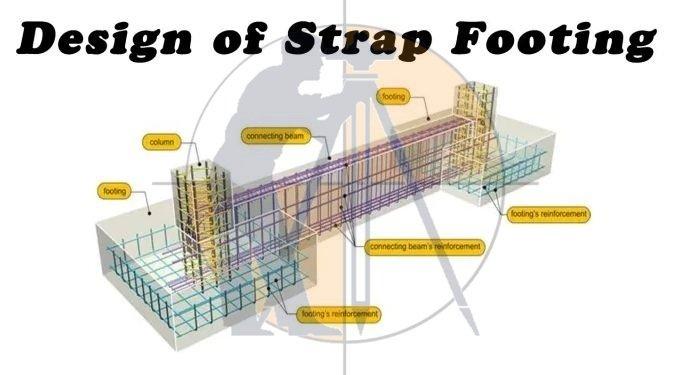### Design assumptions

1. Strap does not provide bearing.
2. Strap is ridge enough to transfer moment from one footing to the other.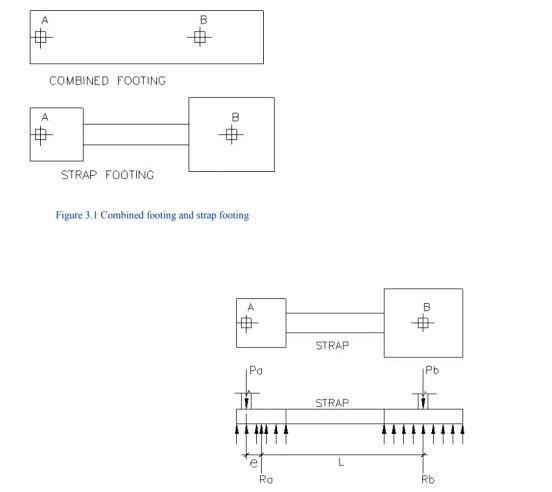### Design procedure

1. Determine the length of exterior footing and its eccentricity, e.
2. Determine eccentric moment, M = Pa*e.
3. Determine shear force, V = M / L
4. Determine footing reaction, Ra = Pa+V, and Rb = Pb-V
5. Determine footing sizes for both A & B.

### Structural analysis

1. Calculate factored column loads, Pua & Pub
2. Calculate factored eccentric moment Mu = Pua*e
3. Calculate factored shear, Vu = Mu / L
4. Determine factored reactions, Rua & Rub.
5. Perform structural analysis, determine factored shear and moment on footings and strap.

### Reinforced concrete design

1. Design exterior footing. Check shear stresses and design flexural reinforcement.
3. Design footing strap as a reinforced concrete beam.

### Design procedure:

1. Determine the length of exterior footing and its eccentricity, e.
2. Determine eccentric moment, M = Pa*e.
3. Determine shear force, V = M / L
4. Determine footing reaction, Ra = Pa+V, and Rb = Pb-V
5. Determine footing sizes for both A & B.

### Column information:

Distance between two columns: 22 ft.

### Footing information:

Allowable soil bearing capacity; 3000 psf

Distance from column A to edge of footing: 1 ft.

Allowable soil bearing capacity = 3000 psf

Weight of soil above footing = 120 psf

Depth of footing= 24”

Depth of soil above footing = 12”

Requirements: Determine the size of footing A & B.

### HOW TO CALCULATE STEEL QUANTITY FOR SLAB WITH BAR BENDING SCHEDULE

Solution: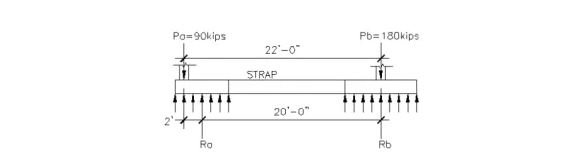Assume a footing width of 6 ft, the eccentricity of footing A is e = 6/2-1=2’.

The distance between footing reaction, L = 22-2=20’

The eccentric moment is M = (40+50)*2=180 ft-kips

The shear produced by M is, V = 180/20=9 kips

Reaction at footing A = 40+50+9 =99 kips

Net soil bearing capacity = 3000-2*150-120=2580 psf

Required footing area of A = 99/2.58=38.4 ft2.

Use 6’ by 6.5’ footing, area = 39 ft2.

Reaction at footing B = 100+80-9=171 kips

Required footing area = 171/2.58=66.3 ft2.

Use 8’ by 8.5’ footing, A = 68 ft2.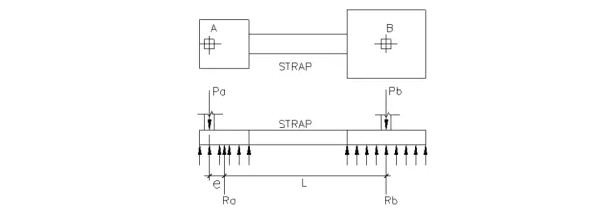### Procedures

1. Calculate factored column loads, Pua & Pub
2. Calculate factored eccentric moment Mu = Pua*e
3. Calculate factored shear, Vu = Mu / L
4. Determine factored reactions, Rua & Rub.
5. Determine factored footing reactions.
6. Perform structural analysis; determine factored shear and moment

on footings and strap.

### Determine moment and shear in a strap footing

Given: The strap footing in

Requirement: Determine maximum factored shears and moment in the footings and strap.

### Solution:

Factored column load of A = 1.4*50+1.7*40=138 kips

### River Sand Vs M Sand | Which Sand is Better For Construction

Factored column load of B = 1.4*100+1.7*80=276 kips

Factored eccentric moment, Mu = 138*2=276 ft-kips

Factored shear, Vu = 276/20=13.8 kips

Factored footing reaction at A = 138+13.8=151.8 kips

Factored footing pressure per linear foot of A = 151.8/6=25.3 k/ft

Factored footing reaction at B = 276-13.8=262.2 kips

Factored footing pressure per linear foot at B = 262.2/8=32.8 k/ft.

### Shear diagram:

At point 1: Vu = 25.3*1.5-138= -100.1 kips

At point 2: Vu = 25.3*6-138=13.8 kips

At point 3: Vu = 25.3*6-138=13.8 kips

At point 4: Vu = 13.8+32.8*3.5= 128.6 kips

At point 5: Vu = 32.8*-3.5=-114.8 kips

### Moment diagram:

At point 1: Mu = 25.3*1.52/2-138*0.5= -40.5 ft-kips

At point 2: Mu = 25.3*62/2-138*5= -234.6 ft-kips

At point 3: Mu = 25.3*6*(6/2+13)-138*(5+13)=-55.2 ft-kips

At point 4: Mu = 25.3*6*(6/2+13+3.5)-138*(5+13+3.5)+32.8*3.52/2=194 ft-kips

At point 5: Mu = 32.8*3.52/2=200.9 ft-kips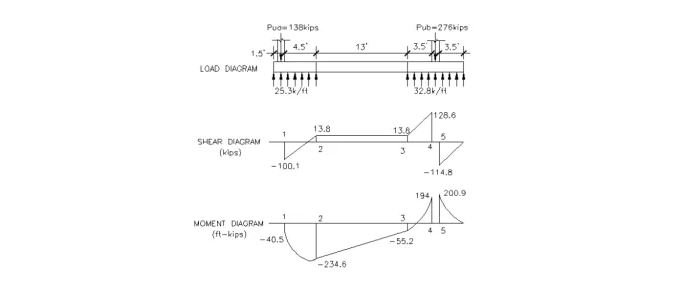### Design procedure:

1. Design exterior footing. Check shear stresses and design flexural reinforcement.
3. Design footing strap as a reinforced concrete beam.

Get Benefits & Share With Others.

### THANKS.